# Auto Highlight Active Row and Column

This post will guide you how to highlight the active row and column in Excel. How do I highlight row and column of active cell with Excel VBA Macro. How to highlight the active row or column when a cell is selected.

## Auto Highlight Row or Column of Active Cell

When you select one cell and you want the row and column in the active cell to auto-highlight. How to achieve it. You should be write a VBA Macro or user defined function to achieve the result. Just do the following steps:

#1 right click on the sheet tab, and select view code from the popup menu list. And the Visual Basic For Application dialog will open.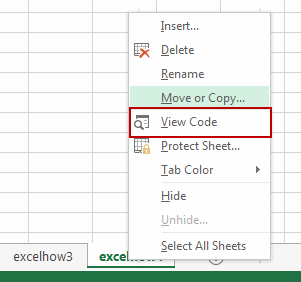#2 type the following VBA Macro into the code window. And save and close the VBA window.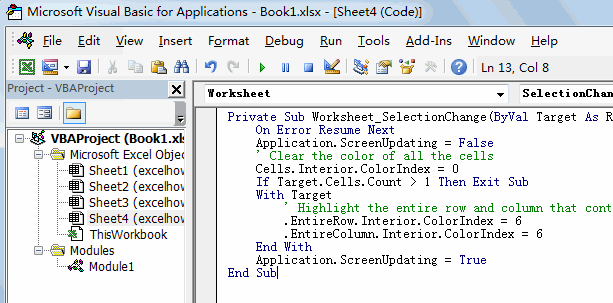```Private Sub Worksheet_SelectionChange(ByVal Target As Range)
On Error Resume Next
Application.ScreenUpdating = False
' Clear the color of all the cells
Cells.Interior.ColorIndex = 0
If Target.Cells.Count > 1 Then Exit Sub
With Target
' Highlight the entire row and column that contain the active cell
.EntireRow.Interior.ColorIndex = 6
.EntireColumn.Interior.ColorIndex = 6
End With
Application.ScreenUpdating = True
End Sub```

#3 select one cell, the entire row and column of active Cell are auto-highlighted.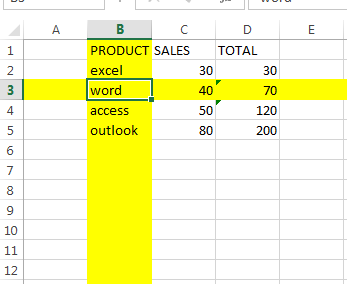## Auto Highlight Active Cell

If you want only to highlight the active cell, you can use the following VBA Macro: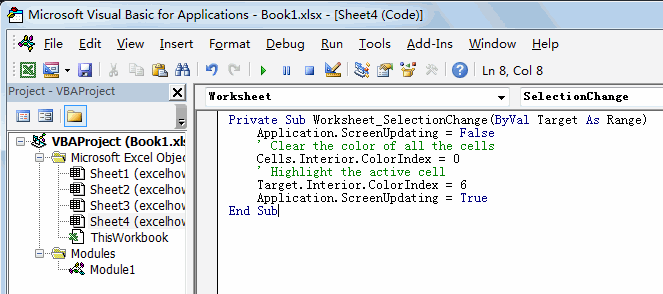```Private Sub Worksheet_SelectionChange(ByVal Target As Range)
Application.ScreenUpdating = False
' Clear the color of all the cells
Cells.Interior.ColorIndex = 0
' Highlight the active cell
Target.Interior.ColorIndex = 6
Application.ScreenUpdating = True
End Sub```

## Auto Highlight Row and Column within the Current Region

If you want to highlight only the row and column within the current region in worksheet, you can use the following VBA Macro: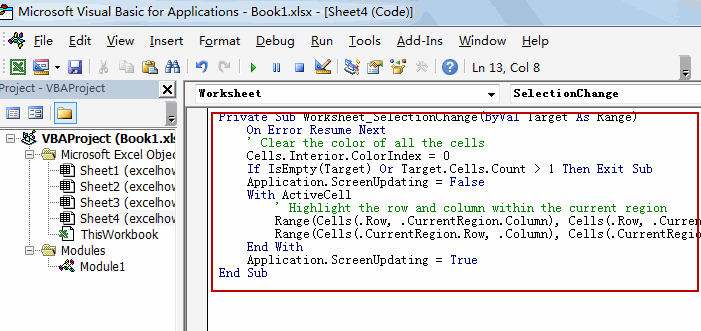```Private Sub Worksheet_SelectionChange(ByVal Target As Range)
On Error Resume Next
' Clear the color of all the cells
Cells.Interior.ColorIndex = 0
If IsEmpty(Target) Or Target.Cells.Count > 1 Then Exit Sub
Application.ScreenUpdating = False
With ActiveCell
' Highlight the row and column within the current region
Range(Cells(.Row, .CurrentRegion.Column), Cells(.Row, .CurrentRegion.Columns.Count + .CurrentRegion.Column - 1)).Interior.ColorIndex = 6
Range(Cells(.CurrentRegion.Row, .Column), Cells(.CurrentRegion.Rows.Count + .CurrentRegion.Row - 1, .Column)).Interior.ColorIndex = 6
End With
Application.ScreenUpdating = True
End Sub```

## Auto Highlight Row and Column with Double-click Cell

If you want to auto-highlight row and column when you double click a cell, you can use the following VBA macro:

```Private Sub Worksheet_BeforeDoubleClick(ByVal Target As Range, Cancel As Boolean)
'Step 1:  Declare Variables
Dim strRange As String
'Step2:  Build the range string
strRange = Target.Cells.Address & "," & _
'Step 3: Pass the range string to a Range
Range(strRange).Select
End Sub```

Related Posts
VBA Macro For VLOOKUP From Another Sheet

In the previous post, you should know that how to fix or remove the #N/A error when using VLOOKUP formula to lookup value from another sheet. And this post will show you how to use VBA code to vlookup data ...

How To Insert Comments in Protected Worksheet in Excel

This post will show you how to allow comments in a protected worksheet in Excel. You can easily to insert comments into cells in a normal worksheet in Excel, but if want to insert a comment in a worksheet that ...

How To Convert Text to Upper Cases(Using VBA) in Excel

This post will show you how to switch from lower case to upper case in Excel. and I am going to show you two different ways of converting text to upper cases using formula or VBA macro in Excel 2013,Excel ...

How To Hide Every Other Row in Excel (Using VBA)

This post will show you how to hide alternate rows or columns in Excel or how to hide every third, fourth, fifth row or column in Excel. If you want to hide every other row in your current worksheet, how ...

How to Disable the Save As Prompt in Excel

This post will show you how to use a VBA Macro to save an Excel file and overwrite any existing file without a prompt so that you are going to get the little window that says file already exists do ...

How to Count Cells that Contain even or odd numbers in Excel

This post will guide you how to count the number of cells that contain odd or even numbers within a range of cells using a formula in Excel 2013/2016.How do I count cells that contain odd numbers through the use ...

How to Count Cells that Contain negative Numbers in Excel

This post will guide you how to count the number of cells that contain negative numbers within a range of cells using a formula in Excel 2013/2016.You can count the number of negative numbers in your data using easy functions ...

How to Count Cells Are Not Blank or Empty in Excel

This post will guide you how to count cells that are not blank or empty in a given range cells using a formula in Excel 2013/2016.How do I count the number of cells that are not blank in a particular ...

How to Count Cells Less Than a Specific Value in Excel

This post will guide you how to count the number of cells less than a particular numeric value in a given range cells using a formula in Excel 2013/2016. How do I count cells that are less than a specific ...

How to Count Cells Greater Than a Specific Value in Excel

This post will guide you how to count the number of cells greater than a particular numeric value in a given range cells using a formula in Excel 2013/2016. How do I count cells that are greater than a specific ...

Sidebar